## Mike on MATLAB GraphicsGraphics & Data Visualization

Note

Mike on MATLAB Graphics has been archived and will not be updated.

# Type 15 Convex Pentagon

Like most computer graphics programmers, I've always been fascinated by the different types of regular tilings of the plane. There was recently some very big news (NPR, the Guardian) in the tiling world. Casey Mann, Jennifer McLoud, and David Von Derau have discovered a new convex pentagon that has a regular tiling. This is the fifteenth known convex pentagon. That's news because pentagons are really the only polygon that is still keeping secrets in this area, and it's been giving them up very slowly. The fourteenth was discovered by Rolf Stein way back in 1985.

Let's take a look at this interesting pentagon. Here are the coordinates of the five vertices.

a = [             0,             0];
b = [          -1/4,    sqrt(3) /4];
c = [-(3+sqrt(3))/4, (1+sqrt(3))/4];
d = [-(4+sqrt(3))/4,           1/4];
e = [            -1,             0];


I'm going to store these in a single array like this:

pts1 = [a(1), b(1), c(1), d(1), e(1); ...
a(2), b(2), c(2), d(2), e(2); ...
1,    1,    1,    1,    1];


That will make them easier to operate on, as you'll see in a bit.

But first off, lets draw the pentagon.

m = zeros(1,5);
colors = lines(6);
ax = axes('Position',[0 0 1 1]);
patch(pts1(1,:),pts1(2,:),m,'FaceColor',colors(1,:));
axis off
axis equal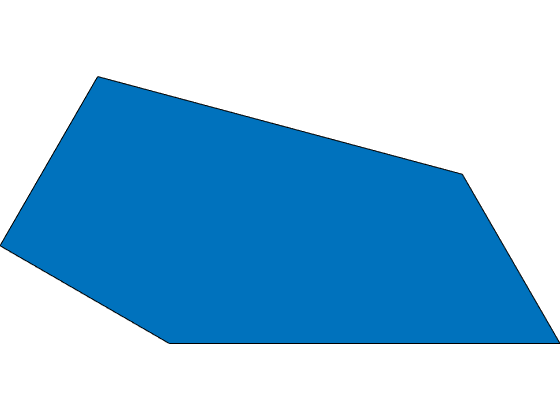Now we can draw a second one by sliding it over to the right and rotating it down.

We do this transformation by creating a 3x3 transform matrix and multiplying the points by it.

To represent a translation in a 3x3 matrix, we have 1's down the diagonal, and our translation vector in the last column.

mat_translate = [1, 0, sqrt(3)/2; ...
0, 1,      -1/2; ...
0, 0,         1];


And a rotation is represented by this familiar matrix:

ang = pi/6;
mat_rotate = [cos(ang), sin(ang), 0; ...
-sin(ang), cos(ang), 0; ...
0,        0, 1];


Now we can multiply pts by the product of those two matrices, like this:

mat = mat_translate*mat_rotate;
pts2 = mat*pts1;
patch(pts2(1,:),pts2(2,:),m,'FaceColor',colors(2,:))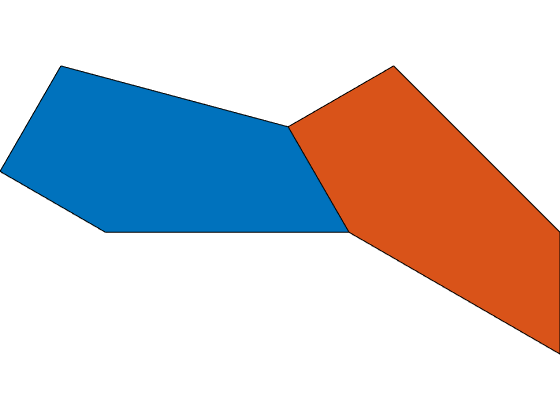We can squeeze a third one in on top of those two, but we need to use a reflection to get a mirror image pentagon. That's allowed in this type of tiling.

A reflection looks like this, when represented as a transform matrix:

mat_reflect = [1,  0, 0; ...
0, -1, 0; ...
0,  0, 1];
mat_translate = [1, 0, (sqrt(3)-1)/4; ...
0, 1, (1+sqrt(3))/4; ...
0, 0,             1];
mat = mat_translate*mat_rotate*mat_reflect;
pts3 = mat*pts1;
patch(pts3(1,:),pts3(2,:),m,'FaceColor',colors(3,:))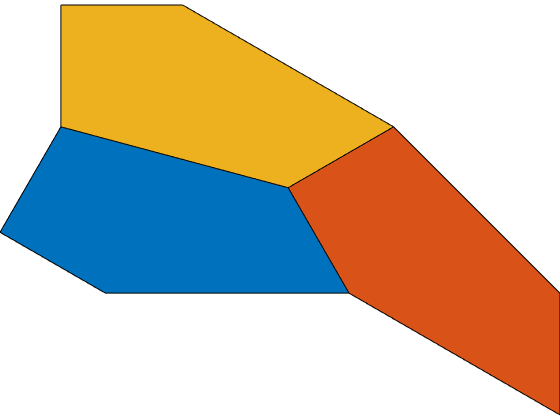Next we can take all three of those and make another reflected and rotated copy.

ang = -pi/3;
mat_reflect = [-1, 0, 0; ...
0, 1, 0; ...
0, 0, 1];
mat_rotate = [cos(ang), sin(ang), 0; ...
-sin(ang), cos(ang), 0; ...
0,        0, 1];
mat_translate = [1, 0, -(5+sqrt(3))/4; ...
0, 1,  (1-sqrt(3))/4; ...
0, 0,              1];
mat = mat_translate*mat_rotate*mat_reflect;
pts4 = mat*pts1;
pts5 = mat*pts2;
pts6 = mat*pts3;
patch(pts4(1,:),pts4(2,:),m,'FaceColor',colors(4,:));
patch(pts5(1,:),pts5(2,:),m,'FaceColor',colors(5,:));
patch(pts6(1,:),pts6(2,:),m,'FaceColor',colors(6,:));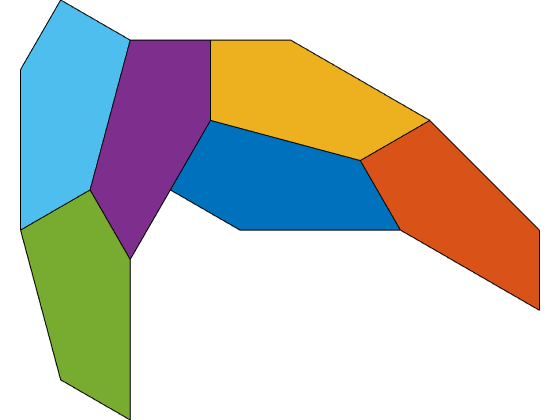And then we can take all six of those, rotate them by pi, and translate them so the two "tails" touch.

ang = pi;
mat_rotate = [cos(ang), sin(ang), 0; ...
-sin(ang), cos(ang), 0; ...
0,        0, 1];
mat_translate = [1, 0, sqrt(3); ...
0, 1,    -1/2; ...
0, 0,       1];
mat = mat_translate*mat_rotate;
next_color = 1;
for p={pts1, pts2, pts3, pts4, pts5, pts6}
tmp_pts = mat*p{:};
patch(tmp_pts(1,:),tmp_pts(2,:),m,'FaceColor',colors(next_color,:));
next_color = next_color+1;
end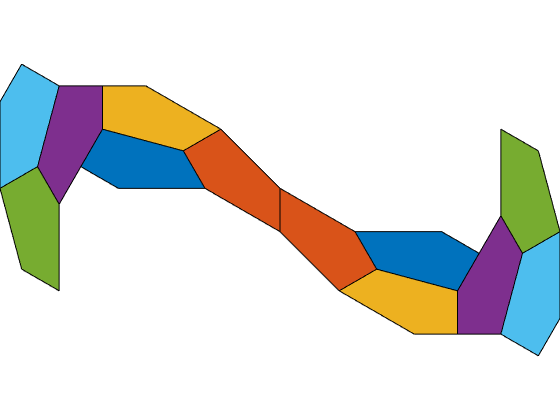This set of twelve pentagons is the fundamental region of the tiling.

We can use these two vectors to place an infinite number of copies of the fundamental set without any gaps or overlaps.

uvec = [  -(1+sqrt(3))/4, (3+  sqrt(3))/4, 0];
vvec = [(10+7*sqrt(3))/4, (3+2*sqrt(3))/4, 0];


Here's the first copy of the fundamental set in the uvec direction. Notice how it tucks up into the corner the first set left.

p = ax.Children;
g = hgtransform;
g.Matrix = makehgtform('translate',uvec);
copyobj(p,g);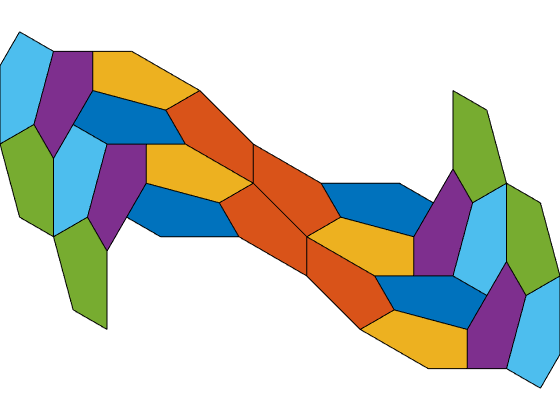And here's the first copy in the vvec direction. It tucks into the V formed by the right hand sides of those first two copies.

g = hgtransform;
g.Matrix = makehgtform('translate',vvec);
copyobj(p,g);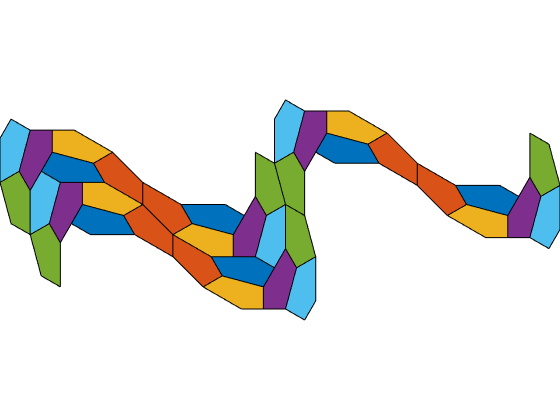If we loop over the two directions, we can see that they do fit nicely, and that you could repeat this pattern forever.

for i=-9:9
for j=-2:2
% Skip the ones we've already drawn
if (i==0 && j==0) || (i==0 && j==1) || (i==1 && j==0)
continue
end
g = hgtransform;
g.Matrix = makehgtform('translate',i*uvec + j*vvec);
copyobj(p,g);
end
end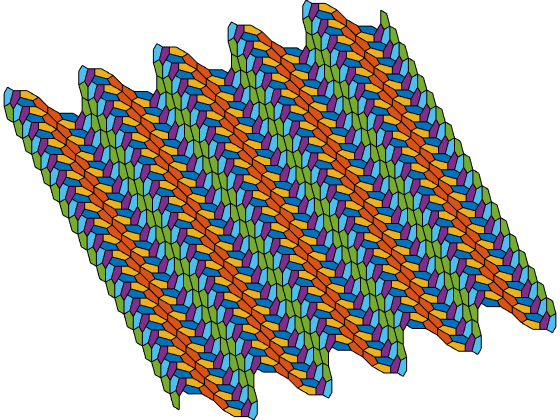Now we'll zoom in to get the full effect.

ylim([-8 8])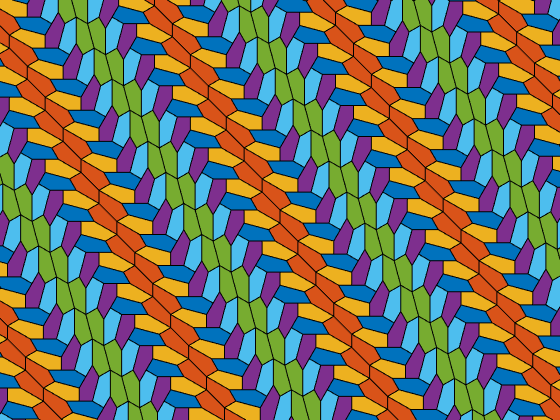Isn't this an interesting tiling? Does it make you want to join the hunt for number sixteen? Do you think number 16 exists?

Published with MATLAB® R2015a